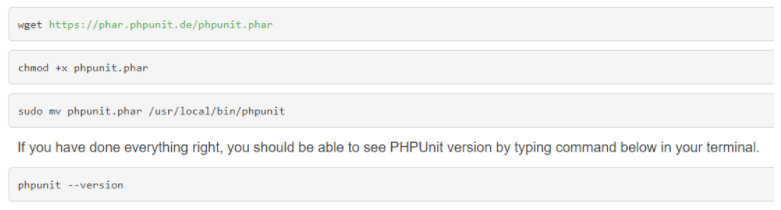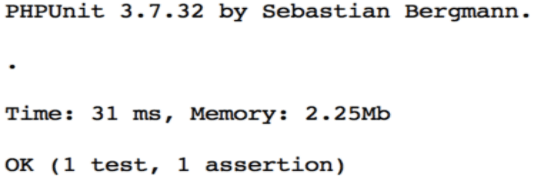Author - StudySection Post Views - 53 views# Software Unit testing in Core PHP

## Introduction

Testing small pieces of code or a particular function or functionality is basically what we call unit testing. Unit test cases should be simple or should not depend on other functions or classes.

The main purpose of unit testing is to make code bug free and also we can detect location like line number and statement of code that is creating problems.

In PHP, we need PHPUnit to run unit test cases. So, follow the below steps to get PHPUnit:### Example to create a unit test

Suppose we have a class like below;

``` <?php Class Calculator{ Public function add(\$x,\$y){ Return \$x+\$y; } } <? ```
Step:1 Create a file named as “CalculatorTest.php”

``` <?php require 'Calculator.php'; class CalculatorTests extends PHPUnit_Framework_TestCase { private \$calculator; protected function setUp() { \$this->calculator = new Calculator(); } protected function tearDown() { \$this->calculator = NULL; } public function testAdd() { \$result = \$this->calculator->add(1, 2); \$this->assertEquals(3, \$result); } } ```
Step:2 Include the class that you want to test.

Step:3 setUp()called before each test case runs.

Step:4 tearDown is similar to setUp() but it runs after each test case runs.

Step:5 testAdd() is the test function for the add() function of Calculator.php because PHPUnit recognizes all the functions with a prefix test as a test function and will run automatically. assertEquals will check if add function returns a correct value.

Finally, PHPUnit CalculatorTest.php to run unit test and you will see the following results:People having good knowledge of Financial accounting can get an Accounting Certification from StudySection to increase their chances of getting a job in this field. You can get a foundation level certification if you are new to Financial accounting or you can go for advanced level certification if you have expert level skills in Financial accounting.